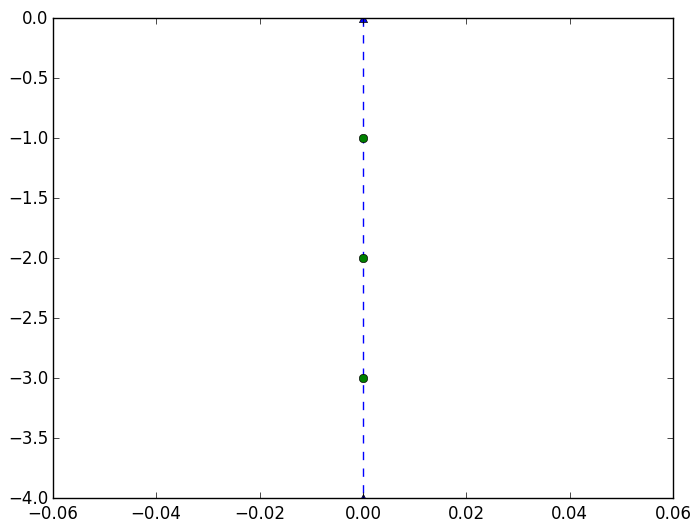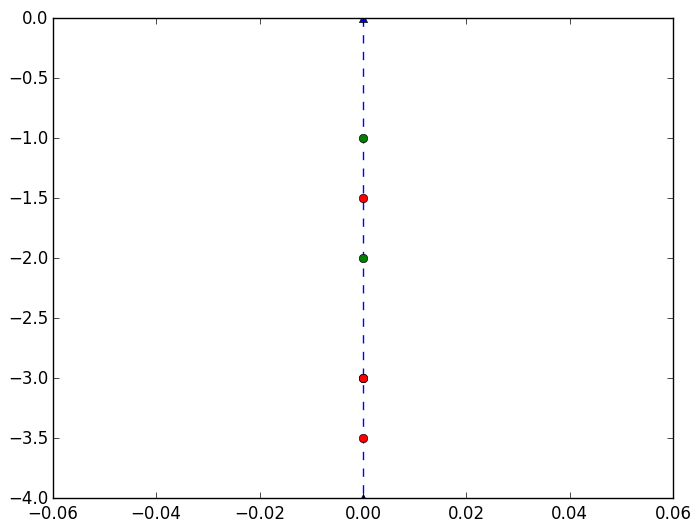# Lecture 6: Linear algebra¶

In this lecture we introduce linear algebra. We first consider the problem of calculating the equilibrium of a system of four springs and three balls, affixed to walls at 0 and 4, where 0 is at the top and 4 is at the bottom.

Here is a plot of our system, where the green circles represent the balls and the blue dotted lines the spring:

In :
using PyPlot  # load the plot command

n=3
plot([0.,0.],[0.,-4.];marker="^",linestyle="--")  # plot triangles to represent the
# anchors at 0 and 4
p=1:n     # The resting position of the balls is equi spaced, so at 1, 2, and 3
plot(zeros(n),-p;marker="o",linestyle="")         # plot the three ballsOut:
1-element Array{Any,1}:
PyObject <matplotlib.lines.Line2D object at 0x3152dfdd0>

Denote the displacement of the balls by $u_1,u_2,u_3$ and the elongation of the springs by $e_1,e_2,e_3,e_4$. Then we have

$$u_1=e_1, u_2 - u_1 = e_2, u_3 - u_2 = e_e, -u_3 = e_4$$

Or in matrix form

$$A\mathbf{u} = \mathbf{e} \qquad\hbox{for}\qquad A = \begin{pmatrix} 1 \cr -1 & 1 \cr &-1 &1 \cr &&-1 \end{pmatrix}, \mathbf{u} = \begin{pmatrix}u_1\cr u_2\cr u_3 \end{pmatrix}, \mathbf{e} = \begin{pmatrix}e_1\cr e_2\cr e_3\cr e_4 \end{pmatrix}$$

Denote the forces of the springs by $y_1,y_2,y_3,y_4$. As the spring becomes more elongated, the force becomes stronger, which we model by Hook's law: $y_k = c_k e_k$ where $c_k$ are the stiffness of each spring. This also can be written in matrix form: $$C {\mathbf e} = {\mathbf y} \qquad \hbox{for} \qquad C = \begin{pmatrix} c_1\cr&c_2\cr&&c_3\cr&&&c_4\end{pmatrix}$$

We finally have the external forces $f_1,f_2,f_3$ pulling down on the balls, these could be gravity or something else. The external force on each ball has to balance with the forces from the spring, giving us:

$$f_1+y_2 = y_1, f_2+y_3 =y_2, f_3 +y_4 = y_3$$

Or in matrix form

$$A^\top \mathbf{y} = \mathbf{f} \qquad \hbox{for} \qquad \mathbf{f} = \begin{pmatrix}f_1\cr f_2\cr f_3\end{pmatrix}$$

We thus want to find the displacement of each ball by solving: $$A \mathbf{u} = \mathbf{e}, C\mathbf{e} = \mathbf{y}, A^\top \mathbf{y} = \mathbf{f}$$ which is equivalent to $$A^\top CAu= f$$ In other words, $$K\mathbf{u} =\mathbf{f}$$ for $K=A^\top C A$.

We will use Julia to solve this equation and plot the results. To do this, we need to create Vectors and Matrices, do Matrix-Matrix multiplication, take transposes and solve linear sytems.

# Creating Vectors¶

The easiest way to create a vector is to use zeros to create a zero Vector and then modify its entries:

In :
v=zeros(5)
v=3.3423
v

Out:
5-element Array{Float64,1}:
0.0
3.3423
0.0
0.0
0.0   

We can specify the type of the Vector by adding it as the first argument to zeros:

In :
v=zeros(Int64,5)
v=3
v

Out:
5-element Array{Int64,1}:
0
3
0
0
0

Note: we can't assign a Float to an integer vector:

In :
v=zeros(Int64,5)
v=3.5

LoadError: InexactError()

in setindex! at array.jl:313

We can also create vectors with ones and rand:

In :
ones(5)

Out:
5-element Array{Float64,1}:
1.0
1.0
1.0
1.0
1.0
In :
ones(Int64,5)

Out:
5-element Array{Int64,1}:
1
1
1
1
1
In :
rand(5)

Out:
5-element Array{Float64,1}:
0.777089
0.75453
0.72074
0.94775
0.842468
In :
rand(Int,5)

Out:
5-element Array{Int64,1}:
-2711362773818306057
4463414055115793868
-4584630395923355567
-6681289071620779384
-6454145051917951527

We have already seen another way to create vectors directly:

In :
[1,2,3,4]

Out:
4-element Array{Int64,1}:
1
2
3
4

When the elements are of different types, they are mapped to a type that can represent every entry. For example, here we input a list of one Float64 followed by three Ints, which are automatically converted to Float64s:

In :
[1.0,2,3,4]

Out:
4-element Array{Float64,1}:
1.0
2.0
3.0
4.0

In the event that the types cannot automatically be converted, it defaults to an Any vector. This is bad performancewise so should be avoided.

In :
[1.0,1,"1"]

Out:
3-element Array{Any,1}:
1.0
1
"1"

We can also specify the type of the Vector explicitly by writing the desired type before the first bracket:

In :
Float64[1,2,3,4]

Out:
4-element Array{Float64,1}:
1.0
2.0
3.0
4.0

We can also create an array using brackets, a formula and a for command:

In :
[k^2 for k=1:5]

Out:
5-element Array{Int64,1}:
1
4
9
16
25
In :
Float64[k^2 for k=1:5]

Out:
5-element Array{Float64,1}:
1.0
4.0
9.0
16.0
25.0

# Creating Matrices¶

Matrices are created similar to vectors, but by specifying two dimensions instead of one. Again, the simplest way is to zeros to create a matrix of all zeros:

In :
zeros(5,5) # creates a 5x5 matrix of Float64 zeros

Out:
5x5 Array{Float64,2}:
0.0  0.0  0.0  0.0  0.0
0.0  0.0  0.0  0.0  0.0
0.0  0.0  0.0  0.0  0.0
0.0  0.0  0.0  0.0  0.0
0.0  0.0  0.0  0.0  0.0

We can also have matrices of different types:

In :
zeros(Int,5,5)

Out:
5x5 Array{Int64,2}:
0  0  0  0  0
0  0  0  0  0
0  0  0  0  0
0  0  0  0  0
0  0  0  0  0

eye creates the identity matrix:

In :
eye(5)

Out:
5x5 Array{Float64,2}:
1.0  0.0  0.0  0.0  0.0
0.0  1.0  0.0  0.0  0.0
0.0  0.0  1.0  0.0  0.0
0.0  0.0  0.0  1.0  0.0
0.0  0.0  0.0  0.0  1.0

We can also create matrices by hand. Here, spaces delimit the columns and semicolons delimit the rows:

In :
[1 2; 3 4; 5 6]

Out:
3x2 Array{Int64,2}:
1  2
3  4
5  6
In :
Float64[1 2; 3 4; 5 6]

Out:
3x2 Array{Float64,2}:
1.0  2.0
3.0  4.0
5.0  6.0

We can also create matrices using brackets, a formula, and a for command:

In :
[k^2+j for k=1:5,j=1:5]

Out:
5x5 Array{Int64,2}:
2   3   4   5   6
5   6   7   8   9
10  11  12  13  14
17  18  19  20  21
26  27  28  29  30

Matrices are really Vectors in disguise. They are still stored in memory in a sequence of addresses. We can see the underlying vector using the vec command:

In :
M=[1 2; 3 4; 5 6]

vec(M)

Out:
6-element Array{Int64,1}:
1
3
5
2
4
6

The only difference between matrices and vectors from the computers perspective is that they have a size which changes the interpretation of whats stored in memory:

In :
size(M)

Out:
(3,2)

Matrices can be manipulated easily on a computer. We can easily take determinants:

In :
M=rand(3,3)
det(M)

Out:
-0.14858065074498047

Or multiply:

In :
M*M

Out:
3x3 Array{Float64,2}:
0.615876  0.457927  0.19322
0.784475  1.06339   0.5475
0.100306  0.403361  0.312523
In :
[1 2; 3 4]*[4 5; 6 7]

Out:
2x2 Array{Int64,2}:
16  19
36  43

If you use .*, it does entrywise multiplication:

In :
[1 2; 3 4].*[4 5; 6 7]

Out:
2x2 Array{Int64,2}:
4  10
18  28

Vectors are thought of as column vectors, and so * is not defined:

In :
a=[1,2,3]
b=[4,5,6]

a*b

LoadError: MethodError: * has no method matching *(::Array{Int64,1}, ::Array{Int64,1})
Closest candidates are:
*(::Any, ::Any, !Matched::Any, !Matched::Any...)
*{T<:Union{Complex{Float32},Complex{Float64},Float32,Float64},S}(!Matched::Union{DenseArray{T<:Union{Complex{Float32},Complex{Float64},Float32,Float64},2},SubArray{T<:Union{Complex{Float32},Complex{Float64},Float32,Float64},2,A<:DenseArray{T,N},I<:Tuple{Vararg{Union{Colon,Int64,Range{Int64}}}},LD}}, ::Union{DenseArray{S,1},SubArray{S,1,A<:DenseArray{T,N},I<:Tuple{Vararg{Union{Colon,Int64,Range{Int64}}}},LD}})
*{TA,TB}(!Matched::Base.LinAlg.AbstractTriangular{TA,S<:AbstractArray{T,2}}, ::Union{DenseArray{TB,1},DenseArray{TB,2},SubArray{TB,1,A<:DenseArray{T,N},I<:Tuple{Vararg{Union{Colon,Int64,Range{Int64}}}},LD},SubArray{TB,2,A<:DenseArray{T,N},I<:Tuple{Vararg{Union{Colon,Int64,Range{Int64}}}},LD}})
...


Whereas entry-wise multiplication works fine:

In :
a.*b

Out:
3-element Array{Int64,1}:
4
10
18

Transposing a Vector gives a row vector, which is represented by a 1 x n matrix:

In :
a'

Out:
1x3 Array{Int64,2}:
1  2  3

Thus we can do dot products as follows:

In :
a'*b

Out:
1-element Array{Int64,1}:
32

This is a vector with one entry, because matrix-vector multiplication always returns a vector. If we use dot, we get the dot product as a scalar:

In :
dot(a,b)

Out:
32

One important note: a vector is not the same as an n x 1 matrix:

In :
v=zeros(Int,3,1)
v[1:3,:]=1:3     # the : notation means all columns

v

Out:
3x1 Array{Int64,2}:
1
2
3
In :
a

Out:
3-element Array{Int64,1}:
1
2
3
In :
v==a

Out:
false

Finally, we can solve linear systems use \:

In :
A=rand(5,5)
b=ones(5)

u=A\b

Out:
5-element Array{Float64,1}:
0.0880726
0.184264
-0.493238
0.606102
1.21375  
In :
A*u-b

Out:
5-element Array{Float64,1}:
0.0
0.0
2.22045e-16
0.0
2.22045e-16

# Back to the spring problem:¶

We now create the relevant matrices and vectors in Julia. We first create A by creating an n+1 x n matrix of zeros, and setting the diagonal to 1 and the subdiagonal to -1:

In :
n=3  # the number of balls

A=zeros(n+1,n)

for k=1:n
A[k,k]=1
A[k+1,k]=-1
end

A

Out:
4x3 Array{Float64,2}:
1.0   0.0   0.0
-1.0   1.0   0.0
0.0  -1.0   1.0
0.0   0.0  -1.0

We now create $C$, where we assume the stiffness is equal. We then can create $K$ and $\mathbf{f}$ and solve the system using \ to find $\mathbf{u}$:

In :
C=eye(n+1)

K=A'*C*A

f=[0,1,0.]

u=K\f  # solves for the displacement u

Out:
3-element Array{Float64,1}:
0.5
1.0
0.5

The masses are now shifted by u. We can plot the new locations in red versus the original locations in green as follows:

In :
plot([0.,0.],[0.,-4.];marker="^",linestyle="--")

p=1:n

newp=p+u

plot(zeros(n),-p;marker="o",linestyle="")
plot(zeros(n),-newp;marker="o",linestyle="")Out:
1-element Array{Any,1}:
PyObject <matplotlib.lines.Line2D object at 0x319ef2f90>

Let's make this example interactive!

In :
using Interact # let's you use @manipulate

In :
n=3  # the number of balls

# set up A
A=zeros(n+1,n)

for k=1:n
A[k,k]=1
A[k+1,k]=-1
end

# the original locations of the springs
p=1:n

# creates a figure to modify
fig=figure()

# @manipulate creates two sliders: one for F and one for c.  F represents the force
#  on the middle ball and c the stiffness of the springs
@manipulate for F=-2.:.01:2., c=1.:10.
withfig(fig) do
C=c*eye(n+1)  # this is the stiffnest matrix

K=A'*C*A

f=[0,F,0.]
u=K\f
newp=p+u

plot([0.,0.],[0.,-4.];marker="^",linestyle="--")
plot(zeros(n),-newp;marker="o",linestyle="")
end
end

Out: